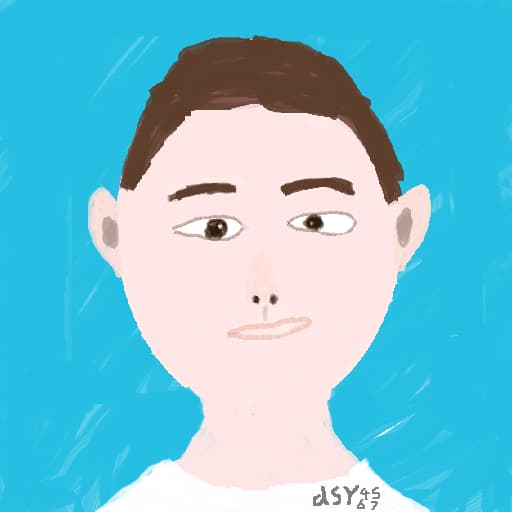# P1932 题解

## 原题

https://www.luogu.com.cn/problem/P1932

``````A+B  A-B  A*B  A/B A%B Problem

# 题目背景

# 题目描述

# 输入格式

A
B

# 输出格式

# 样例 #1

1
1

2
0
1
1
0

# 提示

length(A),length(B)<=10^4

A,B>0

``````

## 代码

``````a,b=int(input()),int(input())
print(a+b)
print(a-b)
print(a*b)
print(a//b)
print(a%b)
``````

Python YYDS!

https://www.luogu.com.cn/record/107080406